# XES - Schema Example

The aim of this page is to propose an XML encoding for source code. This is to enable simpler translation between different language source code, code generators and XMI.

## Variables, declaration and assign.

Variables may be defined, initialised and used in the same statement or in seperate statements. Any variables may also be used in arrays.

All of these things are based on variable node, even assignment will allways have a varible on the left hand side.

When a variable is used we need a quick way to link it back to its definition, how do we define such a link? we need to do this both for local variables, class varibles and varibles in other classes, how do we implement late binding of variables?

Should all node names be expanded out to their full path name?

 Java example XES ```int var1; ```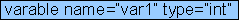`` `var1 = 1;`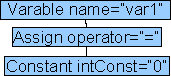``` ``` `int var2 =2;`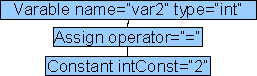`` `int[] array1;`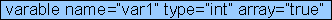`` `array1 = new int;`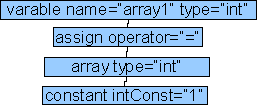``` ``` `array1=3;`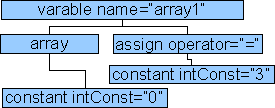``` ``` `int[] array2=new int;```` ``` double x,y,z;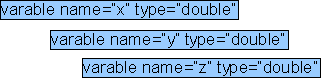`is:` `` `should be:` ```x y z``` JDK 1.5 allows Generic Types: ArrayList list = new ArrayList(); JDK 1.5 also allows enum: public enum StopLight { red, amber, green }; ``` ArrayList ``` ``` StopLight ``` String values[] = (String []) names; (String []) names other possible parameters: type = "=" default type = "++pre" type = "++post" type = "--pre" type = "--post" type = "+=" type = "-=" type = "*=" type = "/=" type = "%=" type = "&=" type = "|=" type = "^=" type = "<<=" type = ">>=" type = "<<<="

## Comment

 Java example XES /* text */ // one line comment text (each line held in separate tags

## Class Declaration

 Java example XES /** class meta description */ class date extends Object { ... } ...parameters ... methods ... other parameters: abstract=true JDK 1.5 allows metadata following @ sign @meta class date extends Object { ... }

## Class Call

this needs more work as class call could occur anywhere in the right hand side of equation

 Java example XES date d = new date(day,month,year);

## Method Declaration

 Java example XES /** move to a point on screen * @param x how far from left of screen * @param y how far from bottom of screen * @returns successful did it work*/ public boolean move(int x,int y){ body } ...body ... other parameters: public=true protected=true private=true final=true synchronised=true native=true Support for javadoc style tags possible tags are: @author classes and interfaces only @version classes and interfaces only @param methods and constructors only) @return methods only) @exception (@throws is a synonym added in Javadoc 1.2) @see @since @serial (or @serialField or @serialData) @deprecated JDK 1.5 allows metadata following @ sign @meta public boolean move(int x,int y){ body }

## Method Call

This is part of assignment (or can be) but I think it is important that method calls are structured so that it is easier to process the structured data to convert to other languages and to make sure that calls link to declarations. So we need to do more work on the structuring of right hand side of equations.

 Java example XES result = move(10,20);

## Package Declare

 Java example XES package myprogram;

## Package Import

 Java example XES import java.io.DataInputStream;

## Interface Declare

 Java example XES /** class meta description */ interface pressEvent { ... } ... methods ... other parameters: abstact=true extends="name" public=true

## Conditionals

 Java example XES if (a==0) else switch (a) { case 0 : ... case 1 : ... default: ... } a==0... ... ... ... ...

## Loops

XES has only one loop type, the java loop types (for, while, do) map to loop by using different combinations of:

• initialise
• operation
• pre-condition
• post-condition

loop has a attribute 'code' which can be (for, while, do) which specifies which combination of (initialise, operation, pre-condition, post-condition) elements are expected between this node. This seems a bit restictave and specific, can anyone think of a better way to specify which subnodes are initialise, operation, pre-condition or post-condition?

 Java example XES ```for (int i=0;i<3;i++) { break; }```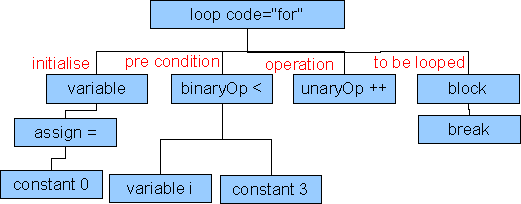``` ``` ```while (true) { break; }```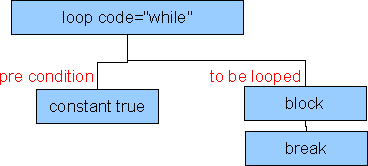``` ``` ```do { break; } while (true);```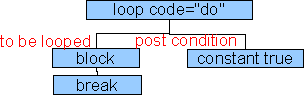``` ``` JDK 1.5 allows enhanced for loop: for (Integer i : list)

## Exceptions

 Java example XES try { } catch () { }